# CBSE Sample Papers for Class 12 Maths UnSolved 2016 Set 7

0
937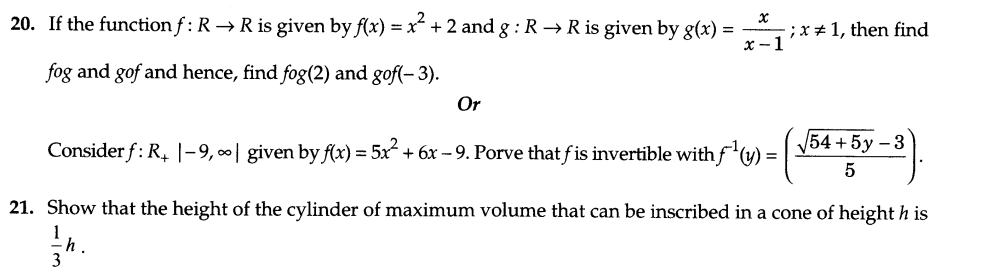SECTION —A

Question numbers 1 to 6 carry 1 mark each.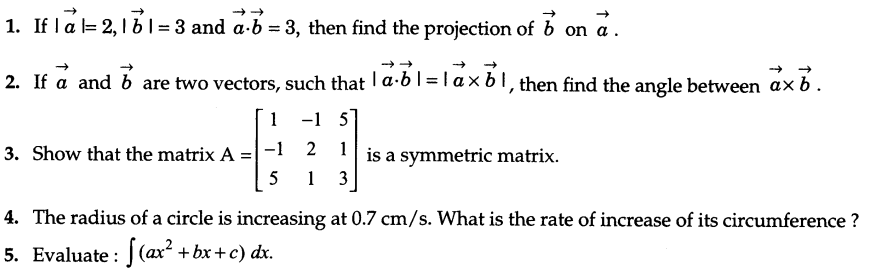6.Find the equation of the plane which is perpendicular to the plane 5x + 3y + 6z + 8 = 0 and which contains the line of intersection of the planes x + 2y + 3z-4 = 0 and 2x + y-z + 5= 0.

SECTION — B

Question numbers 7 to 19 carry 4 marks each.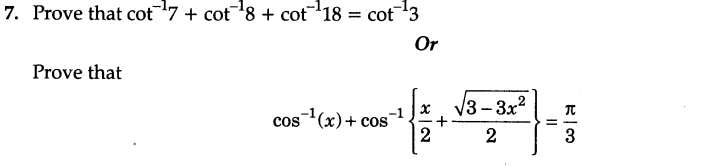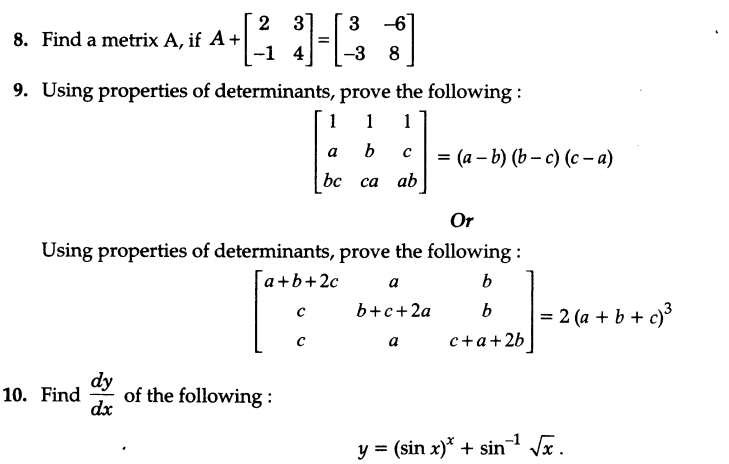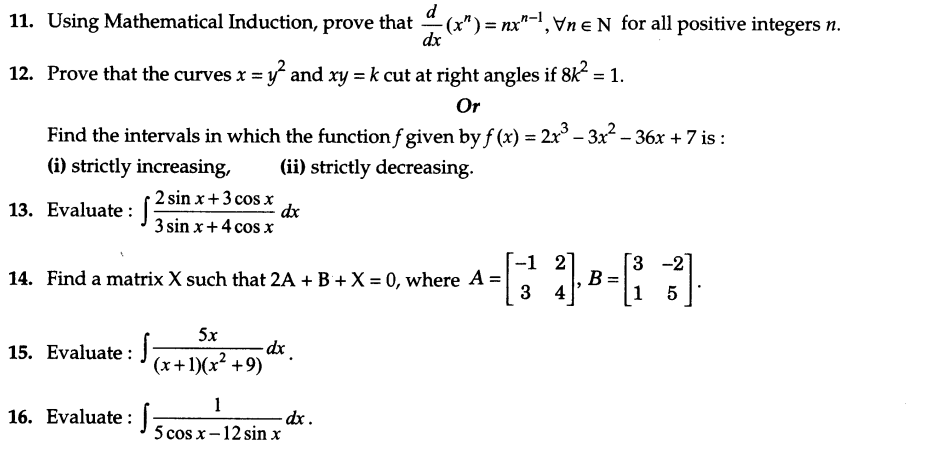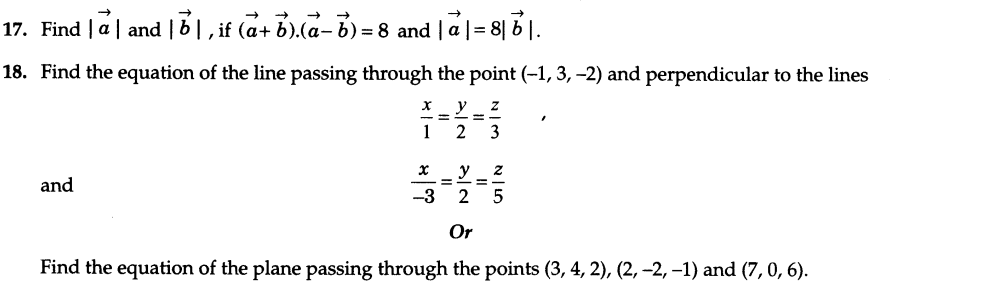19.There are two bags I and II. Bag I contains 3 white and 4 black balls and bag II contains 5 white and 6 black balls. One ball is drawn at random from one of the bags and is found to be white. Find the probability that it was drawn from bag I.

SECTION —C

Question numbers 20 to 26 carry 6 marks each.22.Prove that the curves y2 = Ax and x2=4y divided the area of the square bounded by x = 0, x = 4, y = A and y = 0 into three equal parts.
23.Form the differential equation of the family of circles in the first quadrant which touch the coordinate axes.

24.Find the equation of plane passing through (-1, -1, 2) and perpendicular to each of the following planes :2x + 3y – 3z = 2 and 5x – Ay + z = 6.
Or
Find the vector and cartesian equation of the plane if it meets the x, y and 2 axes respectively such that the centroid of the triangle ABC is (1, -2,3).

25.A farmer has a supply of chemical fertilizer of type I which contains 10% nitrogen and 6% phosphoric acid and type II fertilizer which contains 5% nitrogen and 10% phosphoric acid. After testing the soil conditions of a field, it is found that atleast 14 kg of nitrogen and 14 kg of phosphoric acid is required for a good crop. The fertilizer type I costs Rs 2.00 per kg and the type II costs Rs 3.00 per kg. How many kilograms of each fertilizer should be used to meet the requirement and the cost be minimum ? What value is shown here ?

26.A can hit a target 4 times in 5 shots, B can hit 3 times in 4 shots and C can hit 2 times in 3 shots. Calculate the probability that:
(i) A, B, C all may hit.                                                        (ii) B, C may hit and A may lose.
(iii) Any two of A, B and C will hit the target.              (iv) None of them will hit the target.

Previous articleCBSE Sample Papers for Class 12 Maths UnSolved 2016 Set 6